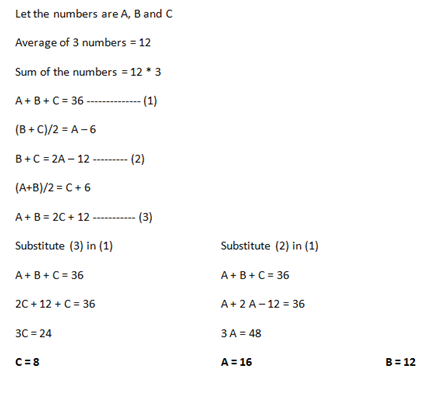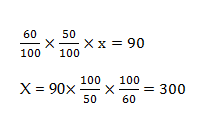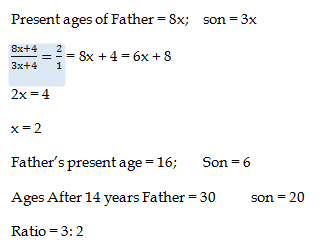# SSC CGL 2018 Practice Test Papers | Quantitative Aptitude (Day-57)

Dear Aspirants, Here we have given the Important SSC CGL Exam 2018 Practice Test Papers. Candidates those who are preparing for SSC CGL 2018 can practice these questions to get more confidence to Crack SSC CGL 2018 Examination.

[WpProQuiz 3694]

Click “Start Quiz” to attend these Questions and view Explanation

1) Average of 3 numbers is 12. First number is 6 more than the average of the other two. Third number is 6 less than the average of other two. What is the difference between first and third number?

a) 8

b) 4

c) 6

d) 10

2) A and B started a business by investing in the ratio 8 : 11. After 6 months, A withdraws his investment. If they receive profits in ratio of 6 : 11, then for how many months was B in the business?

a) 4 months

b) 6 months

c) 8 months

d) 9 months

3) The downstream speed of a boat is 20 kmph and the speed of current is 5 kmph. What will be the total time (in hours) taken by the boat to cover a distance of 150 km in downstream and 100 km in upstream?

a) 15

b) 16. 5

c) 17

d) 17.5

4) The ratio of incomes of Arun and Varun is 11 : 7. If Varun gets Rs.6000 less than Arun, then what is the total income of Arun and Varun?

a) 30000

b) 35000

c) 25000

d) 27000

5) 60 % of 50 % of a number is equal to 90. What is the number?

a) 450

b) 300

c) 540

d) 560

6) How many numbers are there from 150 to 200 which are divisible by 9?

a) 3

b) 6

c) 8

d) 9

7) The selling price of a car is Rs. 450000. If the car is sold at a discount of 10%, then what will be the marked price of the car?

a) 50000

b) 60000

c) 70000

d) 80000

8) How many Numbers are there from 1 to 80 which contains digit 7?

a) 15

b) 17

c) 19

d) 20

9) The ratio of Ravi and his son is 8: 3. After 4 years after the son will be half of his father’s age. What will be the ratio of ages after 14 years?

a) 5 : 2

b) 4: 5

c) 3: 2

d) 5: 7

10) What is the largest three digit number which is exactly divisible by 3, 4 and 5?

a) 910

b) 900

c) 990

d) 960Let A invest 8x and B invest 11x

Investment:        8x × 6                      :           11x × ?

Profit:                  6                              :              11

6 × 8x / 11x = 6 /11

X = 8

B invested his amount in 8 months

Downstream = 20 kmph

Speed of boat = 5 kmph

If the speed of a boat in still water is u km/hr and the speed of the stream is v km/hr, then:

Speed downstream = (u + v) km/hr.

20 kmph = u + 5

Speed of a boat in still water = 15 kmph

Speed of upstream = (u – v) km/hr.

Speed of upstream = 15 – 5 = 10 kmph

Time taken in Upstream = 100 km / 10 kmph

Time taken in downstream = 150 km / 20 km = 7.5 hours

Total time taken = 10 + 7.5 = 17.5 hours

Arun’s income = 11x

Varun’s Income = 7x

11x – 7x = 6000

4x = 6000

x =1500

Total income of Arun and Varun = 11x + 7x = 18x = 18 * 1500 = 27000The numbers which are divisible by 9 = 153, 162, 171, 180, 189, 198

45000*100/90 = 50000

There are 17 digits of 7 from 1 to 80